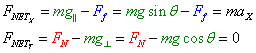# Regents Physics - Ramps and Inclines

## Free Body Diagrams

Now that we've developed an understanding of Newton's Laws of Motion, free body diagrams, friction, and forces on flat surfaces, we can extend these tools to situations on ramps, or inclined, surfaces.The key to understanding these situations is creating an accurate free body diagram after choosing convenient x- and y-axes. Problem-solving steps are consistent with those developed for Newton's 2nd Law.

Let's take the example of a box on a ramp inclined at an angle of Θ with respect to the horizontal. We can draw a basic free body diagram for this situation, with the force of gravity pulling the box straight down, the normal force perpendicular out of the ramp, and friction opposing motion (in this case pointing up the ramp).Once the forces acting on the box have been identified, we must be clever about our choice of x-axis and y-axis directions. Much like we did when analyzing free falling objects and projectiles, if we set the positive x-axis in the direction of initial motion (or the direction the object wants to move if it is not currently moving), the y-axis must lie perpendicular to the ramp's surface (parallel to the normal force). Let's re-draw our free body diagram, this time superimposing it on our new axes.

## Resolving to Components

Unfortunately, the force of gravity on the box, mg, doesn't lie along one of the axes. Therefore, it must be broken up into components which do lie along the x- and y-axes in order to simplify our mathematical analysis. To do this, we can use geometry to break the weight down into a component parallel with the axis of motion (mg) and a component perpendicular to the x-axis (mg) using the equations:Using these equations, we can re-draw the free body diagram, replacing mg with its components. Now all the forces line up with the axes, making it straightforward to write Newton's 2nd Law Equations (FNETx and FNETy) and continue with our standard problem-solving strategy.

In the example shown with our modified free body diagram, we could write our Newton's 2nd Law Equations for both the x- and y-directions as follows:From this point, our problem becomes an exercise in algebra. If you need to tie to two equations together to eliminate a variable, don't forget the equation for the force of friction:.

## Sample Problems

Let's take a look at a sample problem to see how these steps can be put into practice and combined with our knowledge of the kinematic equations:

Let's examine another problem, this time taking a look at a box on a ramp in static equilibrium: